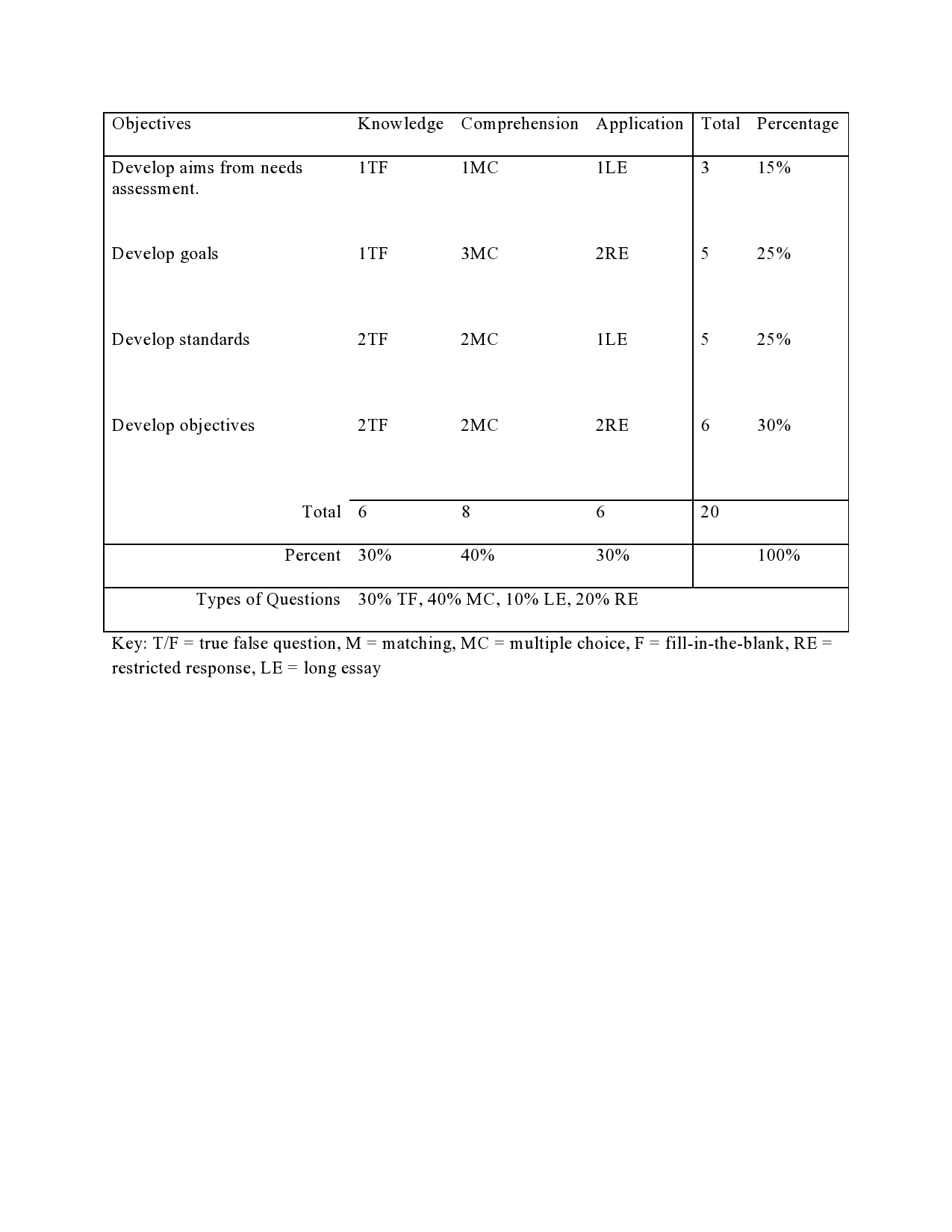# Tag Archives: test blueprint# Test Blueprint

Developing assessments is often difficult. Teachers wonder if they have covered all the material, they have to think about how to assess the students, and they need to consider reaching them at different levels of thinking. This is not in anyway easy for most teachers.

One way to deal with these problems is through the use of what is called a test blueprint. A test blueprint is a map of the objectives that are assessed on the test as well as a map of the different levels of learning that each questionaddresses. Below is an example. You can click on the image to make it bigger.The example above is a test on curriculum development. There are four objectives that are assessed on this test and they are.

1. Develop aims from the needs assessment.
2. Develop goals
3. Develop standards
4. Develop objectives

These four objectives are assessed at one of three levels of Bloom’s taxonomy. These levels are

1. Knowledge
2. Comprehension
3. Application

For these test, I am looking at how my students perform these four objectives at the knowledge, comprehension, and application levels of Bloom’s Taxonomy.

For each level of Bloom’s Taxonomy, I asked 1 or 2 questions related to my objectives. This number is then totaled at the far right. For example, for objective one, developing aims from needs assessment, I have 1 true and false question at the knowledge level, 1 multiple choice question at the comprehension level, and one long essay question at the application level. This gives me a total of 3 questions that focus for the “developing aims from the needs assessment objective.”

These three questions for objective 1 represent 15% of the total questions on the test. In other words, not only do I know how many questions I asked in each level for this objective, I also know how much of the total test is represented in my first objective. This helps in maintaining a balanced test as sometimes teachers give too much weight to one specific piece of information.

Not only can you know how many questions you ask about each objective, you can also know how many questions you have for each level of Bloom’s taxonomy. Forexample, looking at the chart, you can see that there are 6 knowledge questions which represent 30% of the total questions on this exam. Again, such information helps in maintaining a balanced exam.

At the very bottom of the chart, you can also find out how many of each type of question you asked on the exam. For example, there are 6 True and false questions and 8 multiple choice questions for a percentage of 30% and 40% of the total questions. This also helps with balance. You can make sure that different forms of questions are an appropriate percentage of the total exam.

The test blueprint helps teachers to develop balance exams. Objectives, question type, and the level of the questions can all be taken into account when developing an assessment.